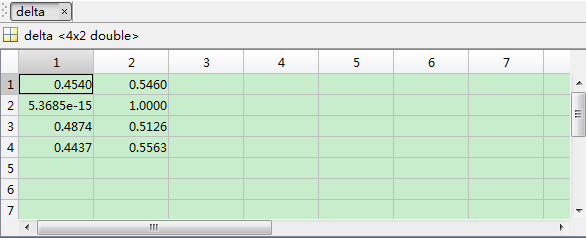# How to assign a value to a cvx variable in the constraints

In my optimization problem, some cvx variables need to assign the exact values, such as:
gamma(5,1) denote the 5*1 variables, and I want to define gamma(2,1)=0 in the constraints. I realize this idea by the following code: gamma(2,1)==0, although the status of result is “solved”, the actual value of gamma(2,1) is not equal to 0. Is it a bug for cvx?

Show your CVX code and results.

Note that the value of gamma(2,1) after solution may not be exactly 0, but only within a tolerance of it. Therefore, after CVX exits, gamma(2,1) == 0 in MATLAB may result in FALSE (0), because gamma(2,1) might be 1e-10, for example. There is no need to see your code if this is all that is happening.

It is not the tolerance problem, if the result is a small value, i can accept it, but the result is around 0.5. Although the cvx get a false result, the status is “solved”, which is confused me.

Unless you show us what you’ve done and your results, I don’t think we can determine anything.

antenna_num = 4;
user_num = 4;
group_num = 2;
sigma = 1;
P = Pmax;
M = neeta;
index=[4,9];
t = length(index);

%% the optimization problem %%%%%%%%%%%
cvx_begin
cvx_solver sdpt3

%% define variables %%%%%%%%%%%
variable W1(antenna_num,antenna_num) hermitian;
variable W2(antenna_num,antenna_num) hermitian;
variable delta(user_num,group_num) nonnegative;

minimize (rou*(trace(W1)+trace(W2)) - sum(sum(delta)));
subject to

%%%% the first constraint %%%%%%%%%%%
gamma(1)*trace(channel1(:,1)*channel1(:,1)’*W2) + gamma(1)sigma <= M(1)(1-delta(1,1)) + trace(channel1(:,1)*channel1(:,1)’*W1);
gamma(1)*trace(channel1(:,1)*channel1(:,1)’*W1) + gamma(1)sigma <= M(1)(1-delta(1,2)) + trace(channel1(:,1)*channel1(:,1)’*W2);

``````     gamma(2)*trace(channel1(:,2)*channel1(:,2)'*W2) + gamma(2)*sigma <= M(2)*(1-delta(2,1)) + trace(channel1(:,2)*channel1(:,2)'*W1);
gamma(2)*trace(channel1(:,2)*channel1(:,2)'*W1) + gamma(2)*sigma <= M(2)*(1-delta(2,2)) + trace(channel1(:,2)*channel1(:,2)'*W2);

gamma(3)*trace(channel1(:,3)*channel1(:,3)'*W2) + gamma(3)*sigma <= M(3)*(1-delta(3,1)) + trace(channel1(:,3)*channel1(:,3)'*W1);
gamma(3)*trace(channel1(:,3)*channel1(:,3)'*W1) + gamma(3)*sigma <= M(3)*(1-delta(3,2)) + trace(channel1(:,3)*channel1(:,3)'*W2);

gamma(4)*trace(channel1(:,4)*channel1(:,4)'*W2) + gamma(4)*sigma <= M(4)*(1-delta(4,1)) + trace(channel1(:,4)*channel1(:,4)'*W1);
gamma(4)*trace(channel1(:,4)*channel1(:,4)'*W1) + gamma(4)*sigma <= M(4)*(1-delta(4,2)) + trace(channel1(:,4)*channel1(:,4)'*W2);
``````

%% the second constraint %%%%%
trace(W1) + trace(W2) <= P;

%% the third constraint %%%%%

``````sum(delta(1,:))<=1;
sum(delta(2,:))<=1;
sum(delta(3,:))<=1;
sum(delta(4,:))<=1;
``````

%% th fourth constraint (actually i want to let the delta(2,1)=0,delta(2,2)=1,and delta(4,1) =delta(4,2)=0) %%%%

for k = 1:t-1
if mod(index(k),group_num) == 0
delta(fix(index(k)/group_num),group_num) == 1;
else
delta(fix(index(k)/group_num)+1,mod(index(k),group_num)) == 1;
end
end

if mod(index(t),group_num) == 0
delta(fix(index(t)/group_num),1) == 0;
delta(fix(index(t)/group_num),2) == 0;
else
delta(fix(index(t)/group_num) + 1,1) == 0;
delta(fix(index(t)/group_num) + 1,2) == 0;
end

%% the fifth constraint %%%%%
W1 == hermitian_semidefinite(antenna_num);
W2 == hermitian_semidefinite(antenna_num);

cvx_end

The following is the result, it can be seen that it is right for delta(2,1) and delta(2,2), but it is false for delta(4,1) and delta(4,2). Are there some thing wrong in my code?I think you are never setting the constraints which you claim are violated. Note that I have not executed your complete code.

t = 2, so mod(index(t),group_num) == 1, so

delta(fix(index(t)/group_num) + 1,1) == 0;
delta(fix(index(t)/group_num) + 1,2) == 0;

should be executed. But fix(index(t)/group_num) + 1 = 5, although delta is declared a 4 by 2 variable. So this should result in a CVX “index exceeds matrix dimensions” error message for you. (If delta were a MATLAB, not CVX, variable, it would create a 5th row of delta.) CVX will continue on and ignore those invalid constraints (delta(5,1) == 0 and delta(5,2) == 0). So it solved a problem, but not the problem you apparently intended.

Look at the output, I think you got the CVX error message which you ignored. You never did set the constraints on delta(4,1) and delta(4,2).

Did you mean to have

delta(fix(index(t)/group_num) ,1) == 0;
delta(fix(index(t)/group_num) ,2) == 0;

i.e., without the “+ 1” ?

1 Like